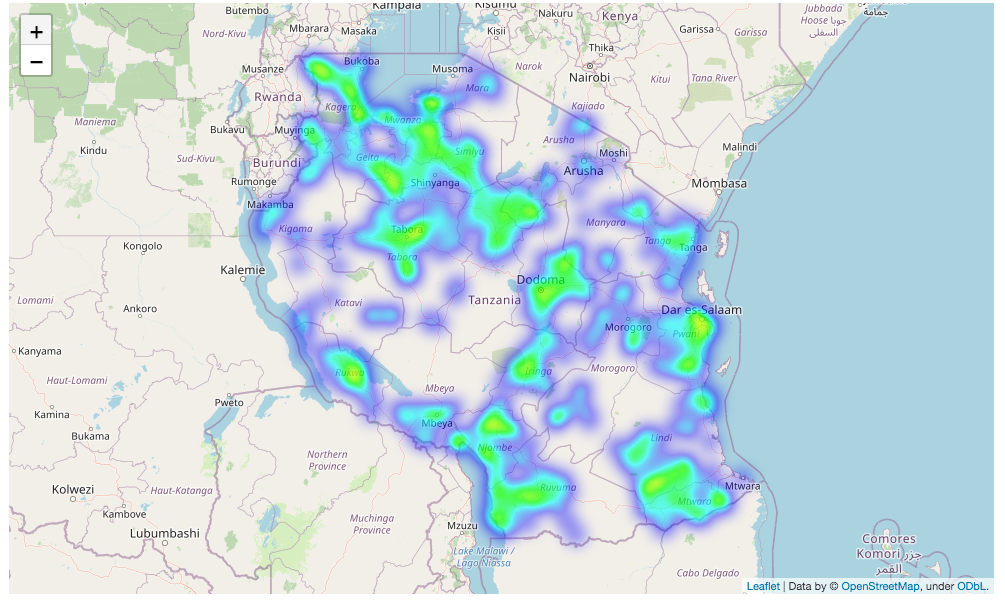# EDA with Folium

An important element for understanding the dataset was the geospatial data for each water point. At the time, I used the Python package Folium to display the water points on a map and filtered the data using different parameters.

`import folium from folium import pluginsimport numpy as npimport pandas as pd# filter the dataframedf = data[data['quantity'] == 'dry']]# create a matrix of data points for the heat maplat_long_matrix = df[['latitude', 'longitude']].to_numpy()# create a folium map centered on Tanzaniamap_ = folium.Map([-6.369, 34.8888], zoom_start=5)# add a heatmap layer the mapmap_.add_child(plugins.HeatMap(lat_long_matrix, radius=10))display(map_)`Water Points with a Quantity value of Dry

# EDA with Tableau

What would have been nice is if I could have made a map like the one above, but that instead of a heatmap, it had color coded marks that gave a easy to understand visualization of the each label for any of the potential values of the quantity parameter. It turns out that is fairly simple to do with Tableau, which can handle mapping larger amounts of data.

# Conclusion

Doing some map based EDA in Tableau allows for a greater understanding of the data than can be done with Python in a Jupyter notebook. The downside is that this part of the EDA is separate from your Jupyter notebooks. Although references and/or screen grabs could be integrated into your notebooks to provide a cohesive story along with your analysis.

--

--## Mike Erb

Data Scientist with a background in Computer Science and as an Entrepreneur in the Bike industry — Based in Ithaca, NY